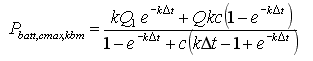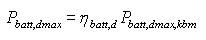HOMER Grid 1.8

 Navigation: HOMER's Calculations How HOMER Calculates the Maximum Battery Discharge PowerIn each time step, HOMER calculates the maximum amount of power that the storage bank can discharge. It uses this "maximum discharge power" when making decisions such as whether the Storage Component can serve the load on its own. The maximum discharge power varies from one time step to the next according to its state of charge and its recent charge and discharge history, as determined by the Kinetic Storage model.

As described in the Kinetic Storage model section of the help, the maximum amount of power that the storage bank can discharge over a specific length of time is given by the following equation:where: Q1 = the available energy [kWh] in the storage at the beginning of the time step Q = the total amount of energy [kWh] in the storage at the beginning of the time step c = the storage capacity ratio [unitless] k = the storage rate constant [h-1] Δt = the length of the time step [h]

HOMER assumes that the discharging losses occur after the energy leaves the two-tank system, hence the storage bank's maximum discharge power is given by the following equation:where ηbatt,d is the storage discharge efficiency.

Modified Kinetic Model

In the Modified Kinetic Battery Model, the losses are modeled with a series resistor. The output power for a given current, I, is defined by the following relation:

Pout = V0I - R0I2

In the above equation, Pout is the output power, V0 is the nominal voltage, and R0 is the series resistance. Therefore, R0I2 is the loss in the resistor. The circuit behavior also leads to a maximum possible output power. At higher currents, the I2 term begins to dominate, and the output power actually decreases with increasing current. We can find the current at this point by setting the derivative dPout/dI to zero:

IPout,max = V0 / (2 R0)

For the Modified Kinetic Battery model, this limit applies at all times, as it does for the Kinetic Battery model limit described in the previous section.